# IONIC BOND

The ionic bond is the electrostatic force of attraction between two oppositely charged ions i.e., a positively charged cation and a negatively charged anion. It is formed due to complete transfer of electrons from one atom to another atom.

The steps involved in the formation of ionic bond can be summarized as:

a) An electropositive atom (metal) loses electron(s) to form a positively charged ion called as cation.

b) An electronegative atom accepts the electron(s) to form a negatively charged ion, otherwise known as anion.

c) Thus formed oppositely charged ions come closer to each other due to electrostatic force of attraction and get stability.

The formation of ionic bond between two atoms can be visualized as follows:* An ionic bond is formed when the electronegativity difference between two bonding atoms is greater than 1.7 on Pauling's scale.

* It can be observed that an ionic bond is formed between an electropositive metal atom and an electronegative nonmetal atom.

KOSSELL'S ELECTRONIC THEORY OF CHEMICAL BONDING

The formation of ionic bond was explained by Kossell as follows:

* The atoms of inert gases are stable due to octet configuration (ns2np6) in their outer shell. Hence atoms must possess eight electrons in their outer shell to get more stability. This is referred to as octet rule

* However, Helium is also highly stable due to 1s2 configuration.

* Therefore every atom tries to get nearest inert gas configuration either by losing or gaining electrons to form ionic bond.

* Only the electrons in the outer shell participate in bond formation. These electrons are also called as valence electrons.

The electrons in the inner shell are called as core electrons and do not participate in the bond formation.

Electrovalency: The number of valence electrons either lost or gained by an atom during the ionic bond formation is called electrovalency.

ILLUSTRATIONS

1) LiF (Lithium fluoride):

* In the formation of Lithium fluoride, the Lithium atom loses one electron to get nearest inert gas: Helium's configuration, 1s2

* Whereas, the Fluorine atom gains one electron to get nearest inert gas: Neon's configuration, 1s22s22p6

* Thus formed Li+ and F- ions get attracted towards each other to form the ionic compound LiF.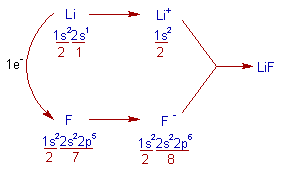* The electrovalency of Lithium is one since it loses one electron.

* The electrovalency of Fluorine is also one since it gains one electron.

Note: The electronegativity difference between Li (1.0) and F (4.0) is 3.0, which is greater than 1.7. Hence they form ionic bond.

2) MgCl2 (Magnesium chloride):

* The Magnesium chloride is an ionic compound. The formation of ionic bond in this compound can be explained as follows.

* The Magnesium atom loses two electrons to get Neon's configuration, [He]2s22p6

* Each Chlorine atom gains one electron to get Argon's configuration, [Ne]3s23p6

* Thus formed Mg2+ and two Cl- ions combine together by forming MgCl2.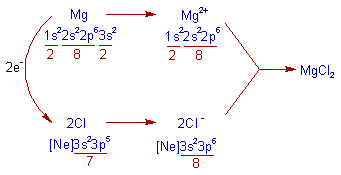* The electrovalency of Mg is 2 and that of Cl is 1.

Note: The electronegativity difference between Mg (1.2) and Cl (3.0) is 1.8. Hence they form ionic bond.

3) AlF3 (Aluminium Fluoride) :

* The Aluminium atom loses 3 electrons and each Fluorine atom gains 1 electron to get their nearest inert gas: Neon's configuration, [He]2s22p6

* Thus formed Al3+ and three F- ions combine together to give AlF3.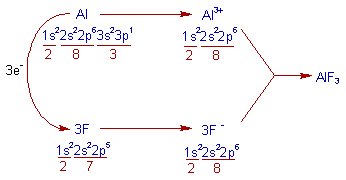* The electrovalency of Al is 3 and that of F is 1.

Note: The bond between Al (e.n = 1.5) and F (e.n = 4.0) is ionic since the electronegativity difference between them is 2.5.

4) Na2O (Sodium monoxide) :

* In the formation of Sodium monoxide, the each sodium atom loses 1 electron and oxygen gains 2 electrons. While doing so, both atoms will get the nearest inert gas: Neon's configuration, [He]2s22p6.

* Thus formed two Na+ and O2- ions combine to form Na2O.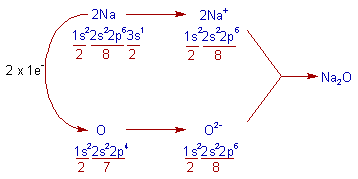* The electrovalency of 'Na' is 1 and that of 'O' is 2.

Note: The bond between Na (e.n = 0.9) and O (e.n = 3.5) is ionic since the electronegativity difference between them is 2.6.

FACTORS FAVORING THE FORMATION OF IONIC BOND

In general, the bond between two atoms gets ionic nature when the electronegativity difference between them is large (greater than 1.7 on Pauling's scale). Since the Ionic bond is electrostatic force of attraction between cation and anion, the factors favouring their formation also favour the formation of ionic bond.

Factors favouring the formation of cation :

1) Large atomic size : In larger atoms, the nuclear attraction over the outer electrons is less. Hence they lose electrons readily to form cations.

E.g. The ease of formation of cation increases from Li+ to Cs+ in IA group with increase in size.

2) Low ionization energy : The removal of electrons is easy from atoms with low ionization energy values. Hence these atoms form cation easily.

E.g. IA and IIA group elements readily form cations due to low ionization energies.

3) Low charge on cation : Since the successive ionization energies are increased (i.e., from IE1 to IE2 to IE3_ _ _), the formation of cations with high charge require more energy. Hence the cations with low charge are formed easily, whereas those with high charge are formed with difficulty.

E.g. Among Na+, Mg2+ and Al3+ , the order of ease of formation is: Na+ > Mg2+ > Al3+

i.e., Na+ is formed more readily than Mg2+ and Al3+

4) Octet electronic configuration : The cations with 8 electrons in the outer shell (octet configuration) are highly stable and hence are formed readily. Whereas cations with 18 electrons in outer shell (Pseudo inert gas configuration) are comparatively less stable and hence are not formed easily.

E.g. The electronic configuration of Ca2+ is [Ne]3s23p6. There are 8 electrons in the outer shell (octet configuration) Hence it is formed easily.

Whereas the electronic configuration of Zn2+ is [Ne]3s23p63d10 (pseudo inert gas configuration). Hence Zn2+ is formed less easily than Ca2+

Factors favouring the formation of anion :

1) Small atomic size : Atoms with small atomic size attract electrons strongly and hence can form anions readily.

2) High electron affinity and electronegativity : The atoms, with high electron affinity and electronegativity, gain electrons easily and hence form anions readily.

E.g., The halogens (VIIA group elements) can form anions readily due to small size, high electron affinity and high electronegativity.

3) Low charge on anion : The formation of highly charged anions is difficult since the addition of successive electrons to the atoms becomes difficult due to repulsion from electrons within the atom. Hence anion with low charge is formed readily.

E.g., Among C4-, N3-, O2- and F- ions, the anion with low charge i.e., F- is formed readily, whereas the formation of anion with higher charge (C4-) is difficult.

i.e., The order of ease of formation of these anions is: C4- < N3- < O2- < F-

PROPERTIES OF IONIC COMPOUNDS

1) Physical state and properties: The ionic compounds contain oppositely charged ions which are strongly attracted to each other. Hence these are hard substances existing as crystalline solids at room temperature. Their melting and boiling points are very high due to strong electrostatic forces of attractions between the ions.

2) Direction of bond: The ionic bond is direction less and the electrostatic forces of attraction are present in all directions around an ion. Hence there is no isolated discrete molecule in the ionic crystal. Entire crystal is considered as the giant molecule.

The ionic compounds do not exhibit isomerism due to non directional nature of ionic bond.

3) Electrical conductivity: In the solid state, the ions cannot move freely and hence they do not conduct electricity. However in the fused state or in aqueous solutions, the ionic compounds show electrical conductivity due to presence of freely moving ions.

4) Solubility: Ionic compounds are polar in nature and hence they are soluble in polar solvents like water. However these are not soluble in non polar solvents like benzene, carbon tetrachloride etc.

5) Reactivity: The reactions between ionic compounds in aqueous solutions occur very fast due to presence of separated ions.

e.g. A white precipitate of AgCl is formed instantly when aqueous solution of NaCl and AgNO3 are mixed.

Formula weight: The ionic compounds contain only ions which are attracted towards oppositely charged ions in all directions. There are no discrete molecules in it. Hence their molar mass is denoted as formula weight instead of molecular weight.

ENERGETICS OF FORMATION OF IONIC COMPOUNDS

The major steps likely to be involved in the formation of ionic compounds can be summarized as:

Formation of gaseous atoms: The atom that is participating in the ionic bond formation is to be isolated from other atoms. This is achieved by converting the metal into gaseous state from either solid or liquid state by supplying energy.In case of non metals, which usually exist in molecular form, an additional step of conversion of gaseous molecules into atoms is required. The energy required for this process is known as bond dissociation energy.Note: Depending on the state of metal or non-metal under the reaction conditions, all of these steps may or may not be required.

Formation of cation from gaseous electropositive atom: A cation is formed when electron(s) are removed from the atom by supplying energy. The minimum amount of energy required for this process is called ionization energy.Formation of anions from gaseous electronegative atom: An anion is formed when electron(s) are added to the atom. In this step, energy is liberated. This is called electron gain enthalpy or electron affinity.Formation of ionic crystal from gaseous ions: The gaseous cations and anions come closer to each other due to electrostatic forces of attractions and form ionic crystal along with the liberation of energy, which is otherwise called as lattice energy.The amount of energy required during the initial steps like fusion, vaporization, sublimation, bond dissociation, removal electrons etc., is usually more than compensated partly by electron gain enthalpy and mostly by lattice energy.The amount of energy liberated i.e. lattice energy will be greater if the attraction between the oppositely charged ions is stronger.

Greater the lattice energy, greater is the ease of formation of that ionic compound. The magnitude of lattice energy depends on the charge density on ions and the distance between them.

Greater the charge density on the ion, greater is its attraction with oppositely charged ions.

Shorter the distance between ions, stronger is the attraction and hence greater is the lattice energy.

Lattice energy of crystal (U): The amount of energy liberated when one mole of the crystalline substance is formed from the constituent gaseous ions is called lattice energy (U) of the crystal.

The determination of lattice energy of NaCl by using Born-Haber cycle is shown below.

CALCULATION OF LATTICE ENERGY OF NaCl: BORN-HABER CYCLE

The principle involved in Born-Haber cylcle is Hess's law of constant heat summation which can be stated as follows:

Hess's Law : The total energy change in a reaction remains same whether the reaction takes place in one step or in several steps.

The NaCl crystals can be obtained from sodium metal and chlorine gas either in one step or in several steps indirectly as shown below.

Direct step : One mole of NaCl crystals are formed by combining one mole of Na metal with half mole of chlorine gas in one step. The energy evolved during this reaction is called heat of formation, ΔHfIndirect method : The formation of NaCl crystals may occur in several steps as follows.

i) Sublimation of sodium : One mole of solid sodium is first converted gaseous sodium by absorbing 108.7 kJ/mole of energy. This is called sublimation energy represented by ΔHs or S.ii) Ionization of sodium : Gaseous sodium atoms are ionized by absorbing 492.82 kJ/mole of energy. It is called ionization energy (ΔHi or I).iii) Dissociation of chlorine molecule : One mole of gaseous chlorine molecules are dissociated into two moles of chlorine atoms by absorbing energy equal to 239.1 kJ/mole. This is called dissociation energy (ΔHd or D).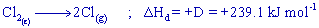But the energy required to get one mole of chlorine atoms is equal to:i.e.,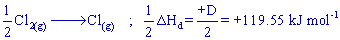iv) Chloride ion formation : The gaseous chlorine atoms are added with electrons to get gaseous chloride ions. The energy liberated in this process is called electron affinity (ΔHe or E).v) Formation of NaCl Crystals : The gaseous Na+ and Cl- ions unite to form one mole of NaCl crystals. The energy liberated during this process is called lattice energy (ΔHu or U).The lattice energy, U can be calculated by using Hess's law as follows:

According to Hess's law, the energy change in the direct step is equal to the sum of energy changes that occur in steps involved in indirect method.orBORN LANDE EQUATION

The lattice energy of an ionic crystal is equal to the sum of attractive and repulsive forces in the crystal. It can be calculated by using Born Lande equation as follows:Where

A = Madelung constant (which depends on geometry of the crystal)The repulsion coefficient depends on the structure and approximately proportional to the number of nearest neighbours

Z+ & Z- = Charges on the positive and negative ions respectively.

e = Charge of an electron.

r = Distance between the oppositely charged ions

n = Born exponent (a constant which is usually taken as 9)

Born Lande equation can be written as follows: